﻿ Biological Models to Study Reaction Kinetic MechanismsPublications are Open
Access in this journal
Article Versions
Export Article
• Normal Style
• MLA Style
• APA Style
• Chicago Style
Research Article
Open Access Peer-reviewed

### Biological Models to Study Reaction Kinetic Mechanisms

Olaf A. Runquist, Bruce M. Boman
World Journal of Chemical Education. 2021, 9(1), 22-27. DOI: 10.12691/wjce-9-1-4
Received January 02, 2021; Revised January 21, 2021; Accepted January 29, 2021

### Abstract

Our goal is to show how modeling of the dynamics of biological behavior in a system of living organisms illustrates the kinetics of molecular reactions. The experiments presented here include real-life modeling of the movement of fish in an aquarium tank and passage of fruit flies through a hole in a chamber. The use of these models also shows, by quantifying the movement of constituents in a system, that an equilibrium reaction is not static system. Rather, it is a dynamic system involving two reactions - a forward reaction and a backward reaction between reactants and products that are fluctuating to and fro in concentration. The results show that modeling behavior of fish represents equilibrium in 3D space while modeling movement of fruit flies characterizes an equilibrium in 2D space. The models also illustrate how biological systems can be used to derive rate constant values and energy of activation for rates of a reaction. Finally, our study illustrates how modeling the dynamics of biological systems provides students with an enhanced understanding of the concepts in chemistry and physics that describe the fundamental kinetic nature of our world.

### 1. Introduction

Reaction rate theory was developed a century ago through the work of van't Hoff, Arrhenius, Bodenstein, and Eyring 1, 2, 3, 4 and many other outstanding chemists [reviewed in 5, 6, 7]. This theory enabled chemists and other scientists to understand mechanisms involved in molecular reactions that occur during transformation of reactants to products. While the concepts of reaction rate are theoretical, the underlying rate laws have allowed chemists to accurately determine the rates of most chemical reactions. However, because of the theoretical nature of reaction rate theory, it has not been possible to visualize the movement and interaction of molecules in chemical reactions that show the dynamics described by the theory. In this paper, we describe and compare two biological systems that allow us to see, in real time, the movement of constituents in a dynamic system and to measure the rates of reactions happening in the system. We will first discuss below the basic principles of reaction kinetics that apply to our biological models, and then we will discuss how model results illustrate mechanisms that explain how molecular reactions progress.

### 2. Basic Principles of Reaction Kinetics

This paper deals with a study of dynamic process in biological systems that demonstrate how dynamics of events occur in molecular reactions. Indeed, the study of rates by which constituents change in number in a biological system illustrates the principles of reaction kinetics. The study of rates in biological systems allows one to appreciate reaction rates that occur in molecular reactions as described by the rate law or rate equation. The rate equation relates change in concentration of reaction constituents to time in the progression of a reaction. For the simple reaction A"B, the rate equation describes disappearance of A or appearance of B as: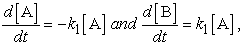(1)

where k is the rate constant and [A] and [B] are concentrations of A and B. If k is known, we can calculate the rate at which the products are produced in a reaction based on concentration of reactants. According to rate law, the value of the rate constant k is temperature dependent and concentrations of reaction constituents are temperature independent.

The rate constant (k) value is determined experimentally during initiation of a reaction, using the integrated version of equation (1):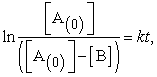(2)

where [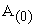] = initial concentration of A and [B] = concentration of B.

This equation has the form of a straight line, so plotting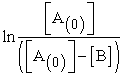versus time (t) gives the rate constant (k) value as the slope of a straight line. Thus, the value of k can be determined for each temperature.

In the progression of reactions, a minimum amount of energy is required in order to activate molecules in transformation of reactants into products. This amount of energy is termed the energy of activation (Ea).

The rate law relationships are described by the Arrhenius equation: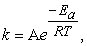(3)

where A = Arrhenius frequency factor, R = molar gas constant (8.3144598 m2 kg s-2 k-1 mol-1), and T = temperature (in degrees Kelvin, oK) .

This equation can be rewritten in the form of a natural logarithm: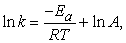(4)

which when plotted will form a straight line.

So, plotting ln k versus 1/T gives -Ea/R as the slope of a straight line and ln A as the intercept.

The above consideration of the fundamental features of reaction rates is also essential to appreciate the dynamics of an equilibrium reaction. The equilibrium reaction is often thought of as a static system in which the concentration of reaction constituents stays constant. But, it is a really dynamic system involving two reactions - a forward reaction and a backward reaction between reactants and products that are fluctuating back and forth in amount. We would like to dispel the notion that equilibrium means that the concentrations of constituents are equal on both sides of the equilibrium. Rather, establishing a dynamic equilibrium means the forward and backward reaction rates are equal. For the simple reaction equilibrium A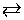B, the rate equation describes changes in concentrations of A and B as: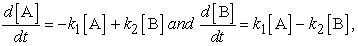Since the forward and the reverse reaction rates are equal, i.e.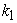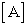=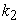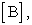the equilibrium constant (Keq) for this reaction is written as: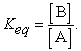So, the condition that has the highest likelihood that [A] = [B] and Keq = 1.0 is when=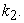But, since a dynamic equilibrium involves backward and forward reactions, the equilibrium constant Keq will not always equal 1.0 when=Thus, consideration of the characteristics of a dynamic equilibrium allows us to appreciate the link between reaction rates and equilibrium.

### 3. Materials and Methods

Experiment 1 (Fish Model). Rate of Minnow Movement in an Aquarium Tank 8.

Studying the dynamic behavior of fish involved measuring the rate at which fish moved in an aquarium tank through the hole in a partition separating one compartment from another compartment. The experimental setup consisted of an aquarium separated into two equal parts by a plastic separator with a central 4 cm diameter hole.

• Aquarium Tank with a Partition

At time zero, a specific number (100-700) of fish was placed in the left compartment of the aquarium. Then a trap door covering the hole was opened and the change in concentration of fish in the right compartment of the aquarium was measured as a function of time. In other words, upon initiation of the experiment, the progression of the reaction was measured as movement of fish (F) from the left (L) compartment into the right (R) compartment (FL " FR). The rate of minnow movement in the tank was also determined at different temperatures and three replicates were run in each experiment.

Experiment 2 (Fruit Fly Model). Rate of Fruit Fly Movement through a Hole 9

The experimental apparatus consisted of a glass tube (50 X 4.6 cm), a Teflon barrier placed in the center, and two movable Teflon pistons. The apparatus was mounted on a board in a horizontal position. A hole in the center of the barrier had a Teflon “trap door”, which could be opened and closed by rotating the apparatus. The movable pistons were perforated to allow passage of air and fitted with thermometers to measure temperatures in each chamber. The surface area of the chambers was determined by a measuring tape on the side of the glass tube.

To conduct an experiment, the “trap door” was closed, the left piston removed and ~100 fruit flies were placed into the left chamber by direct transfer from small breeding bottles (random mixture of males and females of different ages). The piston was quickly replaced and adjusted to the desired position and after a few minutes (allowing the flies to settle down) the “trap door” was opened. The surface concentration of flies in the right chamber was determined at the end of each minute. Alternatively, the number of flies crawling through the hole in the forward and reverse direction per unit time was measured.

Because fruit flies were observed to spend most of their time walking or sitting rather than flying (>95%) and pass through the hole by a crawling (rather than flying) process, the hypothesis was developed 9 that the equilibrium constants for the fruit fly model would be “dependent upon the concentration of flies per unit of surface area rather than volume”. Several experiments were done to test this hypothesis by altering the ratio of volume to surface area in the chambers, so the ratio was not the same in both sides of the apparatus. This was done by inserting thin glass plates into a chamber to adjust the surface area to volume ratio. After the fruit flies were then introduced into the model, a dynamic equilibrium was observed to be established within 1-2 hours. The experiment was repeated at different temperatures where both chambers were maintained at the same temperature. The equilibrium constants were then calculated based upon the concentration of flies in each chamber. Concentrations were calculated in two ways: flies per surface area (cm2) or flies per volume (cm3).

### 4. Results

Experiment 1. Fish Model 8

The rate of minnow movement in the aquarium tank was determined by measuring number of fish in the right compartment as a function of time and temperature (Figure 1A). To determine the rate constant (k) value at each temperature, we used the relationship described by equation (2). Namely, we plotted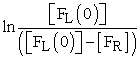versus time (t), where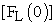= initial concentration of fish in left compartment and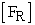= concentration of fish in right compartment. Using this plot (Figure 2A), the rate constant (k) value was determined from slope of the straight line at each temperature. The rate constant (k) values determined from the fish experiments are listed in Table 1. Once we had calculated the rate constant values, we could calculate the energy of activation (Ea) using the Arrenius equation (3). The plot (Figure 3A) of ln k versus 1/T gave us -Ea/R as the slope of a straight line and an energy of activation value of Ea = 52 kJ mol-1.

Experiment 2. Fruit Fly Model 9

The rate of fruit fly movement through a hole was determined by measuring number of fruit flies in the right compartment as a function of time and temperature (Figure 1B). Experimental observation showed that the majority (>95%) fruit flies are not flying at any time and pass through the hole by crawling rather than flying through it. To determine the rate constant (k) value at each temperature, the relationship described by equation (2) was used. Namely, we plotted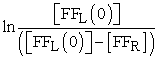versus time (t), where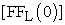= initial concentration of fruit flies in the left compartment and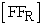= concentration of fruit flies in the right compartment. The rate constant (k) value was determined from slope of the straight line at each temperature (Figure 2B). The rate constant (k) values determined from the fruit fly experiments are listed in Table 2. The energy of activation (Ea) was the determined using the Arrhenius equation (3). The plot (Figure 3B) of ln k versus 1/T gives us an energy of activation value of Ea = 232 kJ mol-1 from the slope of the straight line.

Because the flies were observed to mainly crawl (rather than fly) in the fruit fly model, experiments were done to test the hypothesis that the equilibrium constant is “dependent upon the concentration of flies per unit of surface area rather than volume”. Because the hole in the barrier has parallel sides, the forward and reverse rate constants should be equal and the concentration of flies on both sides should be about the same. In other words, the Keq values should be around 1.0. Table 3 shows that when the volume to surface area is the same on both sides of the apparatus, the equilibrium constants are the same on both sides and the Keq values are about 1.0. In contrast, when the volume to surface areas are not the same on both sides (Table 4), the equilibrium constants are not the same on both sides. So, the Keq value based on flies per surface area is closer to 1.0 compared to the Keq value based on flies per volume. Thus, the results (Table 3, Table 4) show that the Keq values are dependent upon the concentration of flies per unit of surface area rather than volume. This finding established that, in the fruit fly model, the equilibrium constant depends on surface area, rather than volume of the apparatus.

### 5. Discussion

Our comparison of the fish and fruit fly biological systems shows that they have many outstanding features including: 1) the models can easily be built with readily available materials; 2) they allow visualization, in real time, of the movement of the constituents in a dynamic system; 3) the system requires no extraneous energy sources; 4) The rates of reactions happening in the system and rate constants can be measured; 5) the temperature of the model apparatus can be changed so the energy of activation can be determined; 6) The model systems can reach a dynamic equilibrium so equilibrium constants can calculated under different conditions such as varying the surface area as we did in our experiment; 7) The models are structured to maintain constant experimental conditions during each experiment so the results are reproducible and can be statistically analyzed. Several other points bear further discussion on how the models illustrate principles of kinetic reactions.

1. Importance of determining rate constants and energies of activation.

Visualizing the movement of fish and fruit flies over time in these two models allows us to actually see the rate of the reaction as it progresses. Indeed, we observed that the fish swim through the hole faster than the fruit flies craw through the hole. By enumerating the passage of the biologic organisms through the hole, it becomes possible to quantify a rate constant value. Note that the measurement of passage through the hole for fish was plotted in units of seconds (Figure 1A) while for fruit flies it was plotted in minutes (Figure 1B). The corresponding rate constant values for fruit flies was smaller than those for fish, which corresponds with the slower crawling movement of fruit flies than swimming of fish. Then, we showed that the rate constant value is not constant, but is temperature dependent. And, by varying the temperature, determining rate constant values, and doing an Arrhenius plot, we obtained the activation energies. We find that the Ea for the fruit fly model was 4.5-times greater than the Ea for the fish model. This finding illustrates that slow reactions have a large energy of activation, fast ones a small Ea. Moreover, given that the rate constant in the Arrhenius equation is an exponent, relatively small differences in Ea will lead to a large increase in the lifetime (1/k) of the reaction.

2. Significance of the equilibrium constant.

Visualizing the fish and fruit flies also allows us to actually observe the type of movement of constituents in the model. Namely, we saw in the fruit fly model that the flies mainly move by crawling rather than flying in the apparatus. This observation suggested that the spatial dimension of the fruit fly model was not 3D as in the fish model. Accordingly, the dependence of the Keq upon surface area (rather than volume) was investigated in the fruit fly model by inserting thin glass plates into one chamber, and thus, adjusting the surface area to volume ratio. Since the hole in the barrier had parallel sides, all equilibrium constants in the experiment should have had a value around 1.0 as the forward and backward reaction rates should be equal. The results showed that if the volume to surface area in the two chambers is different, the Keq values are dependent upon the concentration of flies per unit of surface area rather than volume. This finding indicated that the spatial dimension of the fruit fly model is 2D rather than 3D. This discovery has several implications and raises several interesting questions that are discussed below.

One implication is that finding that a dynamic equilibrium is established in the 2D fruit fly model as well as in the 3D fish model indicates that the principles of equilibrium and reaction rates are invariant in different spatial dimensions. This idea resonates with the Galilean invariance principle that states the laws of motion are the same in all inertial frames, which was described in 1632. The Galilean invariance principle was a pillar in Albert Einstein’s thought experiment that led to his special theory of relativity.

If rate law and reaction rates are invariant in all dimensions, how do reactions happen in different spatial dimensions? For example, do reactions happen slower and have higher activation energies in 2D compared to 3D systems? How do molecules or particles interact in higher dimensions? These questions may have relevance to string theory that proposes that the Universe consists of point-like particles that can be modeled as one-dimensional objects termed strings. And string theory conjectures that strings propagate through space and interact with each other. Several versions of string theory have been developed and one common feature of the various theories is that they require extra dimensions of space-time to be mathematical consistent. For example, in bosonic string theory, space-time is 26-dimensional, while in superstring theory it is 10-dimensional, and in M-theory it is 11-dimensional 10. However, to model how the strings interact that describes real physical phenomena; it becomes necessary to imagine conditions in which these extra dimensions would not be observed in experiments. Perhaps understanding how reactions happen in different spatial dimensions might provide more insight into how string theory could describe real physical phenomena.

Other questions relating to spatial dimensions arise. Does entropy decrease going from 3D to 2D? Entropy is a state function which relates to any well-defined system at equilibrium. The measure of entropy is often related to density of particles in space. For example, the relation of entropy to spatial dimension (area vs volume) was a consideration by Bekenstein and Hawking in their formulation of an equation for entropy of the event horizon of a black hole. For another example, when an ideal gas is expanded from a volume V to a volume 2V, then the entropy will increase. So, if you have the same number of molecules in a 3D system as in a 2D system, it could be deduced that the molecules will have less space to distributed in 2D than in 3D so the entropy should be lower in 2D. Conversely, does entropy increase in higher dimensions? Thus, it might be conjectured that entropy, like time, is not absolute and is affected by the spatial dimension. Indeed, it is well known that the rate at which time passes depends entirely on speed and acceleration at any given moment.

One might even go so far as to ask - Why is the world we live in three dimensional and not some other spatial dimension? Perhaps if the world was 2D, it has such low entropy that would be so highly ordered that there would be no opportunity for variation. And, conversely, if the world existed in dimensions higher than 3D, perhaps it would be too disordered for structure to evolve. Overall, it is amazing that so many interesting questions were generated simply by comparing two biological models that illustrate principles of rate reactions.

### 6. Conclusions.

The two biological systems presented in this paper allow visualization of the movement of constituents in dynamic system as a reaction progresses. Modeling reactions can make us think of what a rate is and how to define it mathematically. It also makes us appreciate that rate constant and activation matter because they tell us how fast a reaction will happen. Understanding that the rate depends on temperature allows us, by calculating the rate constant at different temperatures, to do an Arrhenius plot that gives us the activation energy 11, 12. And, we find that by doing modeling, more new questions often arise than we have answered. For example, the biological models presented in this paper led to several unanticipated questions including: How do reactions happen in different spatial dimensions. Is entropy, like time, is not absolute and is affected by the spatial dimension. Why is the world we live in three dimensional and not some other spatial dimension? Making changes in these models to test new hypotheses or creating completely new biological models will inspire students to seek answers to even more questions on rates of reaction that catches their imagination.

### References

  van't Hoff, J.H., Études de dynamique chimique, Frederik Muller & Co, Amsterdam, 1884. In article View Article  Arrhenius, S.A., “Über die Dissociationswärme und den Einfluß der Temperatur auf den Dissociationsgrad der Elektrolyte,” Z Phys Chem, 4: 96-116, 1889. In article View Article  Bodenstein, M., “Eine Theorie der photochemischen Reaktionsgeschwindigkeiten,” Z Phys Chem, 85: 390-421, 1913. In article View Article  Eyring, H., “The Activated Complex in Chemical Reactions,” J Chem Phys, 3: 107-115, 1934. In article View Article  Evans, M.G., Polanyi M., “Some applications of the transition state method to the calculation of reaction velocities, especially in solution,” Trans Faraday Soc, 31: 875-894, 1935. In article View Article  Laidler, K., King, C., “Development of transition-state theory,” J Phys Chem, 87: 2657-2664, 1983. In article View Article  Laidler, K.J., Chemical Kinetics, Pearson Education, New Delhi, 2007, 491-520. In article  Runquist, E., Runquist, O.A., “Passage of Fruit Flies through a Hole: A model for a reversible chemical reaction,” J Chem Educ, 49:534-535, 1972. In article View Article  Runquist, O.A., Rates of Reactions in Chemical Principles: An Introductory Programmed Text, Burgess Publishing, Minneapolis, 1974, 382-387. In article  https://en.wikipedia.org/wiki/String_theory [accessed January 24, 2021]. In article  Moore, J.W., Pearson, R.G., Kinetics and Mechanism, John Wiley & Sons, New York, 1981. In article  Sheehan, W.F., Physical Chemistry, Allyn & Bacon, Boston, 1970. In article

Published with license by Science and Education Publishing, Copyright © 2021 Olaf A. Runquist and Bruce M. BomanThis work is licensed under a Creative Commons Attribution 4.0 International License. To view a copy of this license, visit http://creativecommons.org/licenses/by/4.0/